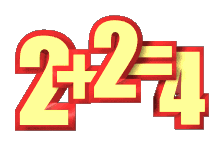MATHEMATICAL ALPHABET LESSON

From a strictly mathematical viewpoint:

What Equals 100%?  What does it mean to give MORE than 100%?

Ever  wonder about those people  who say they are giving more than
100%?

We have all  been in   situations where  someone wants you to give over
100%. How about achieving 101%?  What equals 100% in life?

Here's a little mathematical formula that might help you answer these
questions:

If:
A B C D E F G H I J K L M N O P Q R S T U V W X Y Z

Is represented as:
1 2 3 4 5 6 7 8 9 10 11 12 13 14 15 16 17 18 19  20 21 22 23 24 25 26

Then:

H-A-R-D-W-O-R-K
8+1+18+4+23+15+18+11 =  98%

and

K-N-O-W-L-E-D-G-E
11+14+15+23+12+5+4+7+5 =  96%

But,
A-T-T-I-T-U-D-E
1+20+20+9+20+21+4+5 = 100%

AND,  look how far the love of God will take  you

L-O-V-E-O-F-G-O-D

12+15+22+5+15+6+7+15+4 =  101%

Therefore, one can conclude with mathematical certainty that:

While Hard work and Knowledge will get you close, and Attitude will get
you there, it's the Love of God that will put you over the top!

~author unknown~